Function Repository Resource:

# MeanCI

Estimate the confidence interval for the population mean

Contributed by: Wolfram Research
 ResourceFunction["MeanCI"][list] gives a confidence interval for the population mean estimated from list.

## Details and Options

ResourceFunction["MeanCI"][list] gives a confidence interval {min,max} centered at Mean[list].
If the variance is assumed to be known, ResourceFunction["MeanCI"] is based on the normal distribution.
If the variance is not assumed to be known, ResourceFunction["MeanCI"] is based on the Student's t-distribution with (Length[list]-1) degrees of freedom.
The following options can be given:
 ConfidenceLevel 0.95 probability associated with a confidence interval KnownVariance None variance of population

## Examples

### Basic Examples (1)

A 95% confidence interval for the population mean:

 In:=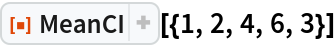Out=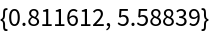### Options (2)

#### ConfidenceLevel (1)

A 99% confidence interval:

 In:=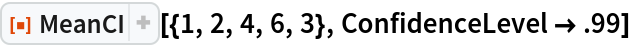Out=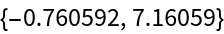#### KnownVariance (1)

A 95% confidence interval assuming the population variance is 1:

 In:=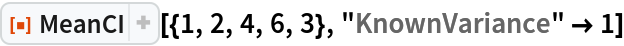Out=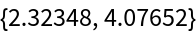## Requirements

Wolfram Language 11.3 (March 2018) or above

## Version History

• 1.0.0 – 13 February 2019